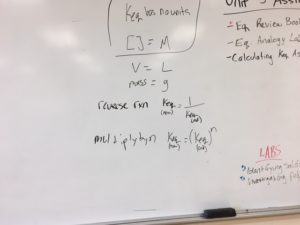Chem 30 Notes

# Sept 27- Calculations using KeqToday we learned about what happens to Keq when you manipulate the chemical reaction. We found that if you reverse the equation, the new keq is the inverse of the original keq. We found if you multiply the equation by some factor (n), you must raise the keq to the power of n.We also performed some calculations using x values as our concentrations.We worked through this powerpoint: lesson 2 2015